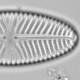## Funky ordination plots with ggvegan

Yesterday, I tweeted a photo of a ordination I plotted with `ggvegan`, and thought I should show how I made it.

Ordinations can be plotted with base R (see `?plot.cca`). This is fine for simple plots, but it is a lot of effort to make a complex plot. The `ggvegan` package, written by Gavin Simpson, lets you use the power of `ggplot2` and can make complex plots easier to make.

Start by loading some packages. `ggvegan` will need to be installed from GitHub if you don’t already have it.

```#load packages
library(tidyverse)
library(vegan)
#devtools::install_github("gavinsimpson/ggvegan")
library(ggvegan)
```

I’m going to use the `pyrifos` dataset, which is included in `vegan`. The data are the abundances of aquatic invertebrates from an experiment in which twelve ditches were studies several times before and after insecticide treatment. We need to make a `tibble` (or a `data.frame`) of the predictors (dose, time and ditch ID).

```#load data
data("pyrifos")

env <- tibble(
week = rep(c(-4, -1, 0.1, 1, 2, 4, 8, 12, 15, 19, 24), each = 12),
dose = factor(rep(c(0.1, 0, 0, 0.9, 0, 44, 6, 0.1, 44, 0.9, 0, 6), 11)),
ditch = gl(12, 1, length=132))
```

We can run a principal components analysis with `rda`.

```pca <- rda(pyrifos)
```

Simple ordination plots can be made with the `autoplot` function. This plot shows all the samples. This is fine, but we want to see dose and time information.

```autoplot(pca, layers = "sites", arrows = FALSE)
```At the moment, it is difficult to do much to this plot. But we can use the `fortify` function from `ggvegan` to extract the scores from the ordination object and then combine these with the predictor data before plotting with `ggplot`. I’m going to colour the samples by dose, and draw a line through the samples in each ditch, with a point on the first value.

```pca_fort <- fortify(pca, display = "sites") %>%
bind_cols(env)

ggplot(pca_fort, aes(x = PC1, y = PC2, colour = dose, group = ditch)) +
geom_path() + #use geom_path not geom_line
geom_point(aes(size = if_else(week == min(week), 1, NA_real_)), show.legend = FALSE) +
scale_color_viridis_d() +
scale_size(range = 2) +
coord_equal()
```Don’t forget to add `coord_equal()` to ensure that the plot is correctly scaled.

Happy plotting.